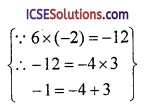The availability of step-by-step OP Malhotra Class 9 Solutions Chapter 4 Factorisation Chapter Test can make challenging problems more manageable.

## S Chand Class 9 ICSE Maths Solutions Chapter 4 Factorisation Chapter Test

Factorise :

Question 1.
8x²y³ – x5
Solution:
8x²y³ – x5 = x²(8y³ – x³)
= x²[(2y)³ – (x)³]
= x²(2y – x) (4y² + 2xy + x²)

Question 2.
x² + $$\frac { 1 }{ x² }$$ + 2 – 2x – $$\frac { 2 }{ x }$$
Solution:
x² + $$\frac{1}{x^2}+2-2 x-\frac{2}{x}$$
= $$\left(x+\frac{1}{x}\right)^2-2\left(x+\frac{1}{x}\right)$$
= $$\left(x+\frac{1}{x}\right)\left(x+\frac{1}{x}-2\right)$$Question 3.
2x² – x – 6
Solution:
2x² – x – 6⇒ 2x² – 4x + 3x – 6
= 2x(x – 2) + 3(x – 2)
= (x – 2) (2x + 3)

Question 4.
a³ – 0.216
Solution:
a³ – 0.216 = (a)³ – (0.6)³
= (a – 0.6) [a² + a x 0.6 + (0.6)²]
= (a – 0.6) (a² + 0.6a + 0.36)

Question 5.
6x²y – xy – 2y
Solution:
6x²y – xy – 2y
= y[6x² – x – 2]
= y[6x² – 4x + 3x – 2]= y[2x(3x – 2) + 1(3x – 2)]
⇒ y(3x – 2) (2x + 1)

Question 6.
(x² – 3x)² – 8(x² – 3x) – 20
Solution:
(x² – 3x)² – 8(x² – 3x) – 20
Let x² – 3x = y,
then y² – 8y – 20⇒ y(y – 10) + 2(y – 10)
⇒ (y – 10) (y + 2)
⇒ (x² – 3x – 10) (x² – 3x + 2)
⇒ {x² – 5x + 2x – 10} {x² – x – 2x + 2}
⇒ {x(x – 5) + 2(x – 5)} {x(x – 1) – 2(x – 1)}
⇒ (x – 5) (x + 2) (x – 1) (x – 2)

Multiple Choice Questions

Question 7.
One of the factors of (x – 1) – (x² – 1) is
(a) x² – 1
(b) x + 1
(c) x – 1
(d) x + 4
Solution:
(c) x – 1
(x – 1) – (x² – 1)
= (x – 1) – (x + 1) (x – 1)
= (x – 1) [1 – X + 1]
∴ x – 1 is its one factor.Question 8.
If $$\frac{x}{y}+\frac{y}{x}$$ = – 1 (x, y ≠ 0), then the value of x³ – y³ is
(a) 1
(b) – 1
(c) $$\frac { 1 }{ 2 }$$
(d) 0
Solution:
(d) 0
$$\frac{x}{y}+\frac{y}{x}$$ = – 1
$$\frac{x^2+y^2}{x y}$$ = – 1 ⇒ x² + y² = – xy
Now, x³ – y³ = (x – y) (x² + xy + y²)
= (x – y) x 0 = 0

Question 9.
The product of $$\left(x-\frac{1}{x}\right)\left(x+\frac{1}{x}\right)\left(x^2+\frac{1}{x^2}\right)$$
(a) x4 + $$\frac{1}{x^4}$$
(b) x³ + $$\frac { 1 }{ x³ }$$ – 2
(c) x4 – $$\frac{1}{x^4}$$
(d) x² + $$\frac { 1 }{ x² }$$ + 2
Solution:
(c) x4 – $$\frac{1}{x^4}$$Question 10.
If x – 2y= 11 and xy = 8, then the value of x³ – 8y³ is
(a) 1860
(b) 1600
(c) 1859
(d) 2000
Solution:
x – 2y – 11, xy = 8
x – 2y = 11
Cubing both sides,
(x – 2y)³ = (11)³
⇒ x³ – 8y³ – 3 × x × (2y) (x – 2y) = 1331
⇒ x³ – 8y³ – 6xy(x – 2y) =1331
⇒ x³ – 8y³ – 6 x 8 x 11 = 1331
⇒ x³ – 8y³ – 528 = 1331
x³ – 8y³ = 1331 + 528 = 1859
∴ x³ – 8y³ = 1859